# 第一次作业。

7-7 寻找250

1.实验代码

#include<stdio.h>
int main()
{
int sum,i=1,n;
scanf("%d",&sum);
if(sum==250)
{
i=1;

}else
{
for(n=1;n<10000;n++)
{
scanf("%d",&sum);
i=i+1;
if(sum==250)
{
break;
}
}
}
printf("%d",i);
}

2.流程图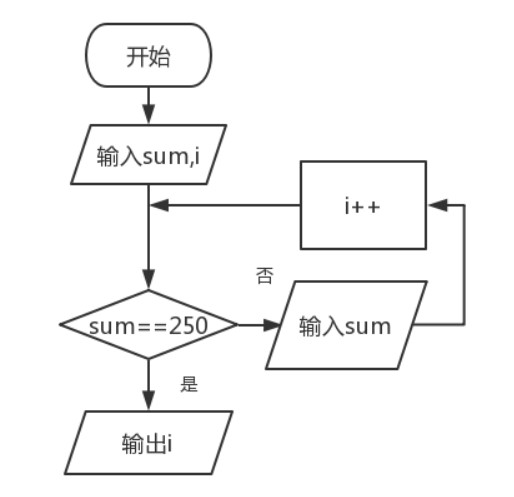3.本题调试过程碰到问题及解决办法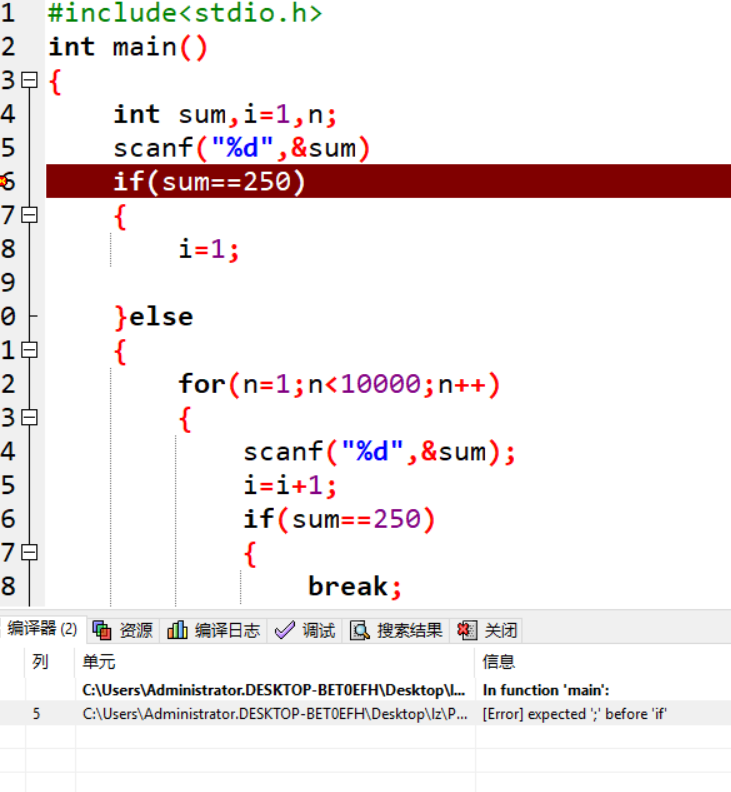3.本题调试过程碰到问题及解决办法

7-6 求1到100的和

1.代码

#include<stdio.h>
main()
{
int i,sum= 0;
for(i = 1;i <= 100;i++)
sum = sum + i;
printf("sum = %d\n",sum);
}

2.流程图

3.本题调试过程碰到问题及解决办法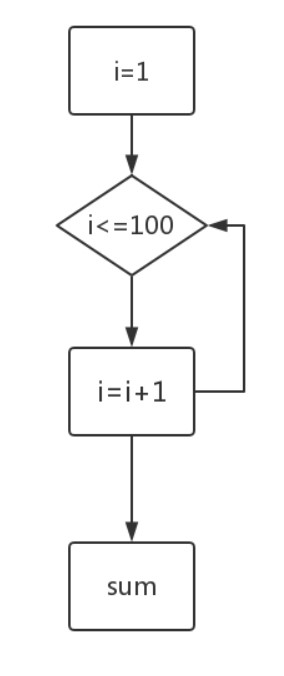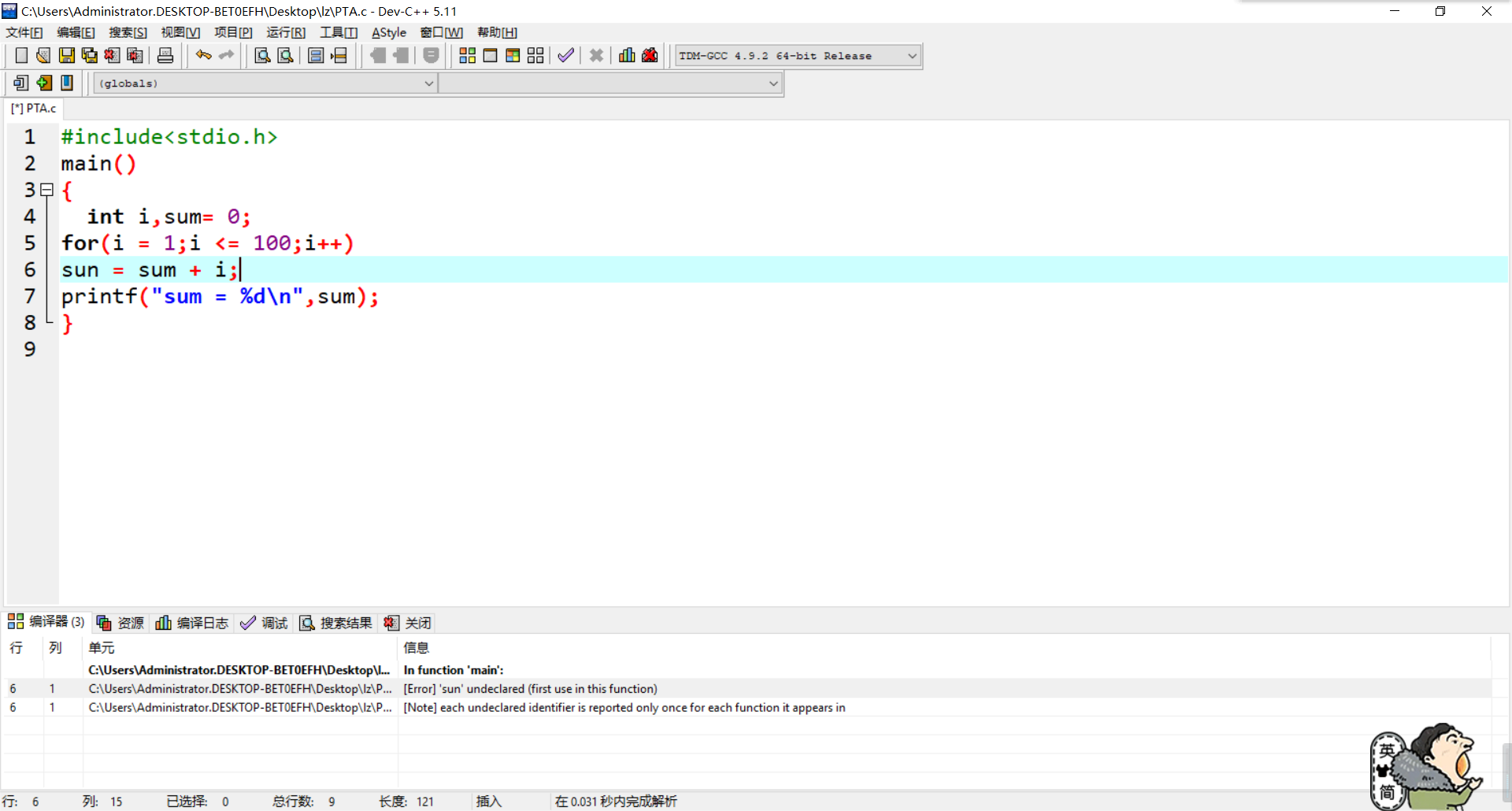计算机18秋_分支结构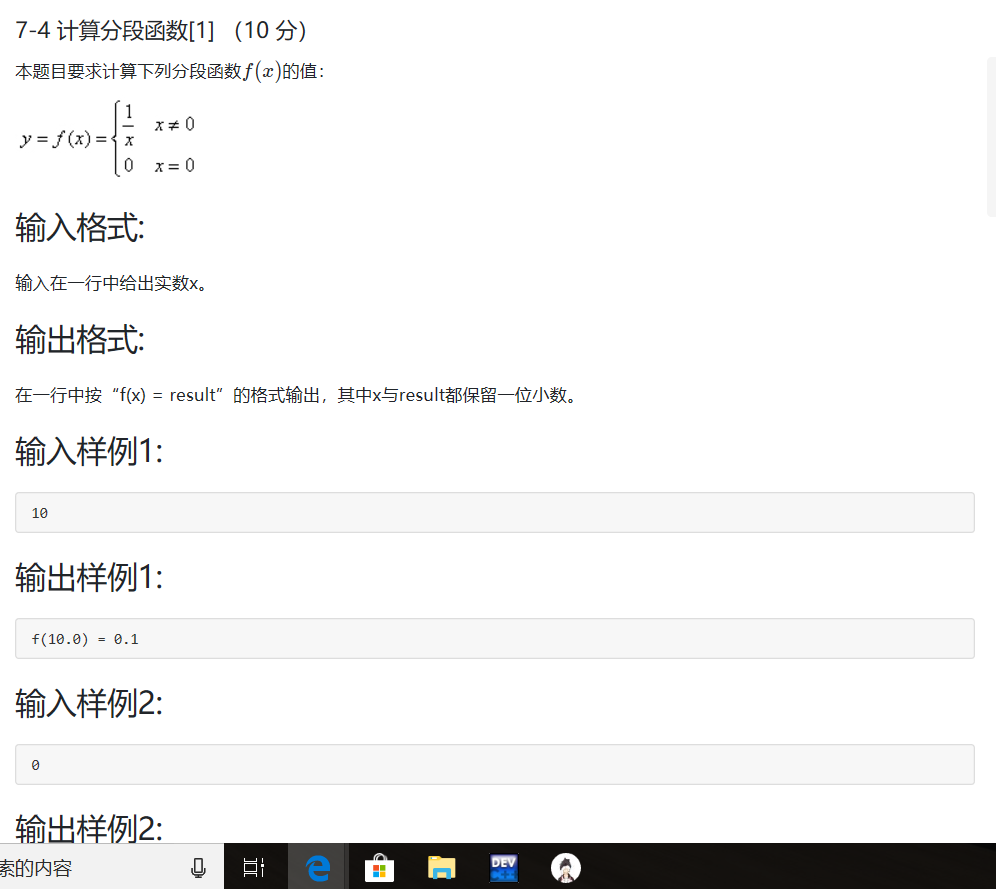1.实验代码

#include<stdio.h>
int main()
{
double x,y;
scanf("%lf",&x);
if(x!=0){
y=1/x;
}else{
y=0;
}
printf("f(%.1lf) = %.1lf",x,y);
return 0;

}


2.流程图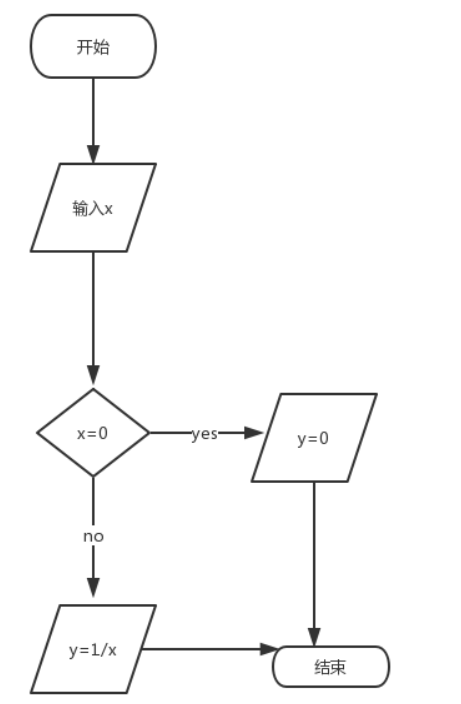3.本题调试过程碰到问题及解决办法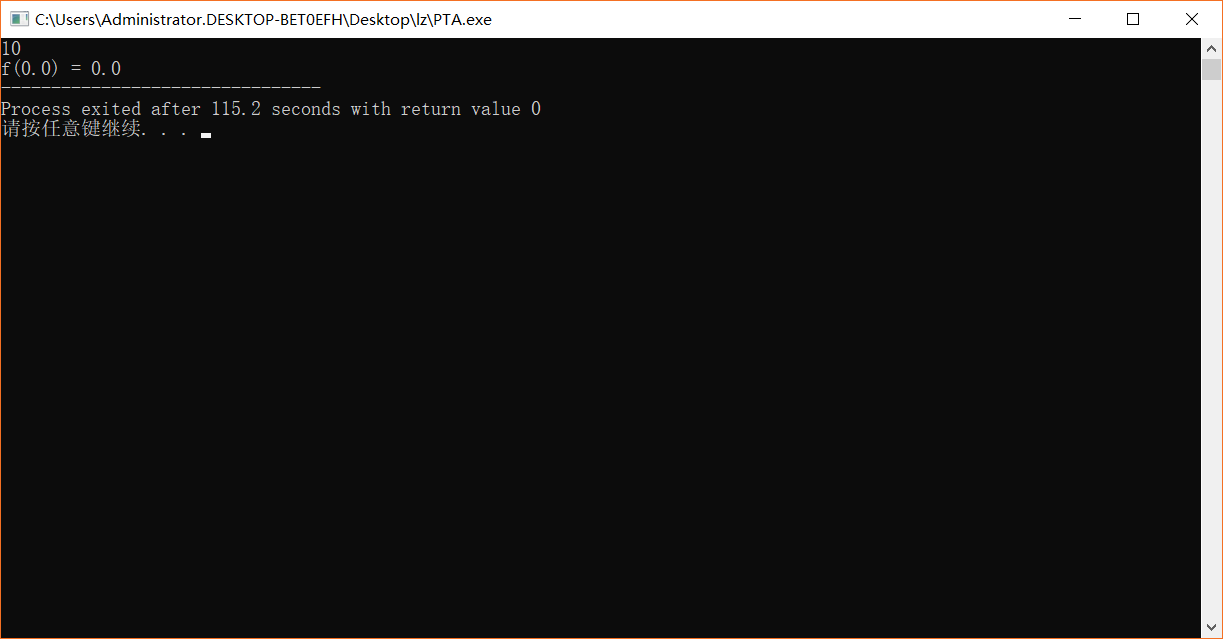3.解决方法

2、点评3个同学的本周作业

https://www.cnblogs.com/mcba22/p/10041195.html孟琛博奥

https://www.cnblogs.com/12921-ly/p/10035869.html#commentform刘洋

https://www.cnblogs.com/zyn-66/p/9984819.html#commentform章宇楠

3.表格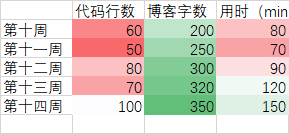4.折线图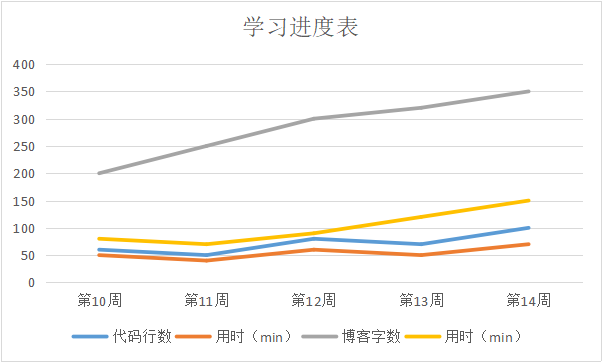posted @ 2018-11-30 18:57  冰心一十月  阅读(207)  评论(13编辑  收藏  举报